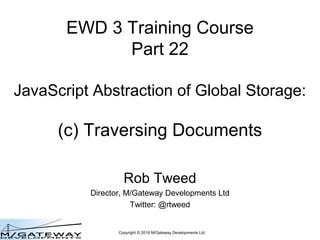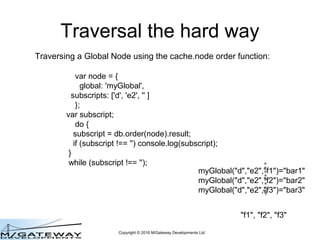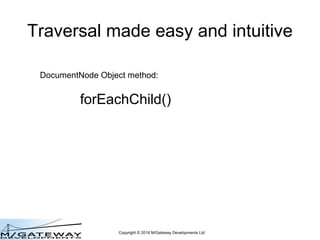Successfully reported this slideshow.

# EWD 3 Training Course Part 22: Traversing Documents using DocumentNode Objects×

1 of 52
1 of 52

# EWD 3 Training Course Part 22: Traversing Documents using DocumentNode Objects

This presentation is Part 22 of the EWD 3 Training Course. It examines how to traverse Documents and navigate within them using DocumentNode methods and properties

This presentation is Part 22 of the EWD 3 Training Course. It examines how to traverse Documents and navigate within them using DocumentNode methods and properties

### EWD 3 Training Course Part 22: Traversing Documents using DocumentNode Objects

1. 1. Copyright © 2016 M/Gateway Developments Ltd EWD 3 Training Course Part 22 JavaScript Abstraction of Global Storage: (c) Traversing Documents Rob Tweed Director, M/Gateway Developments Ltd Twitter: @rtweed
2. 2. Copyright © 2016 M/Gateway Developments Ltd Traversing a Global Node using the cache.node order function: var node = { global: 'myGlobal', subscripts: ['d', 'e2', '' ] }; var subscript; do { subscript = db.order(node).result; if (subscript !== '') console.log(subscript); } while (subscript !== ''); myGlobal("d","e2","f1")="bar1" myGlobal("d","e2","f2")="bar2" myGlobal("d","e2","f3")="bar3" "f1", "f2", "f3" Traversal the hard way
3. 3. Copyright © 2016 M/Gateway Developments Ltd DocumentNode Object method: forEachChild() Traversal made easy and intuitive
4. 4. Copyright © 2016 M/Gateway Developments Ltd forEachChild() Method • Iterates through all child nodes of a DocumentNode Object – ie through subscripts in underlying Global Storage
5. 5. Copyright © 2016 M/Gateway Developments Ltd forEachChild() Method • Iterates through all child nodes – ie through subscripts in underlying Global Storage – docNode.forEachChild(function(nodeName, childNode) {…});
6. 6. Copyright © 2016 M/Gateway Developments Ltd forEachChild() Method • Iterates through all child nodes – ie through subscripts in underlying Global Storage – docNode.forEachChild( function(nodeName, childNode) {…}) ; – Callback function fires on every iteration • ie every time a child node is found
7. 7. Copyright © 2016 M/Gateway Developments Ltd forEachChild() Method • Iterates through all child nodes – ie through subscripts in underlying Global Storage – docNode.forEachChild(function(nodeName, childNode) {…}); Child node's Node Name (ie subscript)
8. 8. Copyright © 2016 M/Gateway Developments Ltd forEachChild() Method • Iterates through all child nodes – ie through subscripts in underlying Global Storage – docNode.forEachChild(function(nodeName, childNode) {…}); Child node's Node Name (ie subscript) DocumentNode object representing the Child node
9. 9. Copyright © 2016 M/Gateway Developments Ltd myDoc("d","e2","f1")="bar1" myDoc("d","e2","f2")="bar2" myDoc("d","e2","f3")="bar3" var e2Node = new this.documentStore.DocumentNode('myDoc', ['d', e2']); forEachChild() Method
10. 10. Copyright © 2016 M/Gateway Developments Ltd myDoc("d","e2","f1")="bar1" myDoc("d","e2","f2")="bar2" myDoc("d","e2","f3")="bar3" var e2Node = new this.documentStore.DocumentNode('myDoc', ['d', e2']); e2Node.forEachChild(function(nodeName) { // do something with the node name / subscript for this iteration }); forEachChild() Method
11. 11. Copyright © 2016 M/Gateway Developments Ltd myDoc("d","e2","f1")="bar1" myDoc("d","e2","f2")="bar2" myDoc("d","e2","f3")="bar3" var e2Node = new this.documentStore.DocumentNode('myDoc', ['d', e2']); e2Node.forEachChild(function(nodeName) { // 1st iteration nodeName === 'f1’ }); forEachChild() Method
12. 12. Copyright © 2016 M/Gateway Developments Ltd myDoc("d","e2","f1")="bar1" myDoc("d","e2","f2")="bar2" myDoc("d","e2","f3")="bar3" var e2Node = new this.documentStore.DocumentNode('myDoc', ['d', e2']); e2Node.forEachChild(function(nodeName) { // 2nd iteration nodeName === 'f2’ }); forEachChild() Method
13. 13. Copyright © 2016 M/Gateway Developments Ltd myDoc("d","e2","f1")="bar1" myDoc("d","e2","f2")="bar2" myDoc("d","e2","f3")="bar3" var e2Node = new this.documentStore.DocumentNode('myDoc', ['d', e2']); e2Node.forEachChild(function(nodeName) { // 3rd iteration nodeName === 'f3’ }); forEachChild() Method
14. 14. Copyright © 2016 M/Gateway Developments Ltd myDoc("d","e2","f1")="bar1" myDoc("d","e2","f2")="bar2" myDoc("d","e2","f3")="bar3" var e2Node = new this.documentStore.DocumentNode('myDoc', ['d', e2']); e2Node.forEachChild(function(nodeName) { console.log(nodeName); }); forEachChild() Method f1 f2 f3
15. 15. Copyright © 2016 M/Gateway Developments Ltd myDoc("d","e2","f1")="bar1" myDoc("d","e2","f2")="bar2" myDoc("d","e2","f3")="bar3" var e2Node = new this.documentStore.DocumentNode('myDoc', ['d', e2']); e2Node.forEachChild(function(nodeName, childNode) { // do something with the childNode DocumentNode object for this iteration }); forEachChild() Method
16. 16. Copyright © 2016 M/Gateway Developments Ltd myDoc("d","e2","f1")="bar1" myDoc("d","e2","f2")="bar2" myDoc("d","e2","f3")="bar3" var e2Node = new this.documentStore.DocumentNode('myDoc', ['d', e2']); e2Node.forEachChild(function(nodeName, childNode) { // do something with the childNode DocumentNode object for this iteration }); forEachChild() Method
17. 17. Copyright © 2016 M/Gateway Developments Ltd myDoc("d","e2","f1")="bar1" myDoc("d","e2","f2")="bar2" myDoc("d","e2","f3")="bar3" var e2Node = new this.documentStore.DocumentNode('myDoc', ['d', e2']); e2Node.forEachChild(function(nodeName, childNode) { // 1st iteration }); forEachChild() Method
18. 18. Copyright © 2016 M/Gateway Developments Ltd myDoc("d","e2","f1")="bar1" myDoc("d","e2","f2")="bar2" myDoc("d","e2","f3")="bar3" var e2Node = new this.documentStore.DocumentNode('myDoc', ['d', e2']); e2Node.forEachChild(function(nodeName, childNode) { // 2nd iteration }); forEachChild() Method
19. 19. Copyright © 2016 M/Gateway Developments Ltd myDoc("d","e2","f1")="bar1" myDoc("d","e2","f2")="bar2" myDoc("d","e2","f3")="bar3" var e2Node = new this.documentStore.DocumentNode('myDoc', ['d', e2']); e2Node.forEachChild(function(nodeName, childNode) { // 3rd iteration }); forEachChild() Method
20. 20. Copyright © 2016 M/Gateway Developments Ltd myDoc("d","e2","f1")="bar1" myDoc("d","e2","f2")="bar2" myDoc("d","e2","f3")="bar3" var e2Node = new this.documentStore.DocumentNode('myDoc', ['d', e2']); e2Node.forEachChild(function(nodeName, childNode) { // do something with the childNode DocumentNode object for this iteration }); forEachChild() Method childNode is a DocumentNode Object, so has all the usual properties and methods
21. 21. Copyright © 2016 M/Gateway Developments Ltd myDoc("d","e2","f1")="bar1" myDoc("d","e2","f2")="bar2" myDoc("d","e2","f3")="bar3" var e2Node = new this.documentStore.DocumentNode('myDoc', ['d', e2']); e2Node.forEachChild(function(nodeName, childNode) { console.log(childNode.value); }); forEachChild() Method bar1 bar2 bar3
22. 22. Copyright © 2016 M/Gateway Developments Ltd forEachChild() Method • Iterates through all child nodes – docNode.forEachChild(function(nodeName, childNode) {…}); • Note that because ewd-document-store uses synchronous versions of underlying cache.node APIs, the call-backs fire in synchronous order • Additionally, the forEachChild() function will wait until completion before next command executes • This greatly simplifies its use!
23. 23. Copyright © 2016 M/Gateway Developments Ltd myDoc("d","e2","f1")="bar1" myDoc("d","e2","f2")="bar2" myDoc("d","e2","f3")="bar3" var e2Node = new this.documentStore.DocumentNode('myDoc', ['d', e2']); console.log('start…'); e2Node.forEachChild(function(nodeName, childNode) { console.log(childNode.value); }); console.log('end'); Example start… bar1 bar2 bar3 end
24. 24. Copyright © 2016 M/Gateway Developments Ltd myDoc("d","e2","f1")="bar1" myDoc("d","e2","f2")="bar2" myDoc("d","e2","f3")="bar3" var e2Node = new this.documentStore.DocumentNode('myDoc', ['d', e2']); console.log('start…'); e2Node.forEachChild(function(nodeName, childNode) { console.log(childNode.value); }); console.log('end'); Example This can be done safely because QEWD uses the master / worker architecture of ewd-qoper8 where your handler functions run on their own in an isolated process
25. 25. Copyright © 2016 M/Gateway Developments Ltd myDoc("d","e2","f1")="bar1" myDoc("d","e2","f2")="bar2" myDoc("d","e2","f3")="bar3" var e2Node = new this.documentStore.DocumentNode('myDoc', ['d', e2']); e2Node.forEachChild(function(nodeName, childNode) { // if callback function returns true (exit boolean flag), it stops the loop }); Stopping forEachChild() Early
26. 26. Copyright © 2016 M/Gateway Developments Ltd myDoc("d","e2","f1")="bar1" myDoc("d","e2","f2")="bar2" myDoc("d","e2","f3")="bar3" var e2Node = new this.documentStore.DocumentNode('myDoc', ['d', e2']); var count = 0; e2Node.forEachChild(function(nodeName, childNode) { count++; if (count === 3) return true; console.log(childNode.value); }); Stopping forEachChild() Early bar1 bar2
27. 27. Copyright © 2016 M/Gateway Developments Ltd myDoc("a")=123 myDoc("b","c1")="foo" myDoc("b","c2")="foo2" myDoc("d","e1","f1a")="bar1a" myDoc("d","e1","f2a")="bar2a" myDoc("d","e2","f1b")="bar1b" myDoc("d","e2","f2b")="bar2b" myDoc("d","e2","f3b")="bar3b" Nested forEachChild() loops Traversal of all nodes and subnodes
28. 28. Copyright © 2016 M/Gateway Developments Ltd myDoc("a")=123 myDoc("b","c1")="foo" myDoc("b","c2")="foo2" myDoc("d","e1","f1a")="bar1a" myDoc("d","e1","f2a")="bar2a" myDoc("d","e2","f1b")="bar1b" myDoc("d","e2","f2b")="bar2b" myDoc("d","e2","f3b")="bar3b" Nested forEachChild() loops Traversal of all nodes and subnodes
29. 29. Copyright © 2016 M/Gateway Developments Ltd myDoc("a")=123 myDoc("b","c1")="foo" myDoc("b","c2")="foo2" myDoc("d","e1","f1a")="bar1a" myDoc("d","e1","f2a")="bar2a" myDoc("d","e2","f1b")="bar1b" myDoc("d","e2","f2b")="bar2b" myDoc("d","e2","f3b")="bar3b" Nested forEachChild() loops Traversal of all nodes and subnodes
30. 30. Copyright © 2016 M/Gateway Developments Ltd myDoc("a")=123 myDoc("b","c1")="foo" myDoc("b","c2")="foo2" myDoc("d","e1","f1a")="bar1a" myDoc("d","e1","f2a")="bar2a" myDoc("d","e2","f1b")="bar1b" myDoc("d","e2","f2b")="bar2b" myDoc("d","e2","f3b")="bar3b" Nested forEachChild() loops Traversal of all nodes and subnodes
31. 31. Copyright © 2016 M/Gateway Developments Ltd myDoc("a")=123 myDoc("b","c1")="foo" myDoc("b","c2")="foo2" myDoc("d","e1","f1a")="bar1a" myDoc("d","e1","f2a")="bar2a" myDoc("d","e2","f1b")="bar1b" myDoc("d","e2","f2b")="bar2b" myDoc("d","e2","f3b")="bar3b" Nested forEachChild() loops Traversal of all nodes and subnodes
32. 32. Copyright © 2016 M/Gateway Developments Ltd myDoc("a")=123 myDoc("b","c1")="foo" myDoc("b","c2")="foo2" myDoc("d","e1","f1a")="bar1a" myDoc("d","e1","f2a")="bar2a" myDoc("d","e2","f1b")="bar1b" myDoc("d","e2","f2b")="bar2b" myDoc("d","e2","f3b")="bar3b" Nested forEachChild() loops Traversal of all nodes and subnodes
33. 33. Copyright © 2016 M/Gateway Developments Ltd myDoc("a")=123 myDoc("b","c1")="foo" myDoc("b","c2")="foo2" myDoc("d","e1","f1a")="bar1a" myDoc("d","e1","f2a")="bar2a" myDoc("d","e2","f1b")="bar1b" myDoc("d","e2","f2b")="bar2b" myDoc("d","e2","f3b")="bar3b" Nested forEachChild() loops Traversal of all nodes and subnodes
34. 34. Copyright © 2016 M/Gateway Developments Ltd myDoc("a")=123 myDoc("b","c1")="foo" myDoc("b","c2")="foo2" myDoc("d","e1","f1a")="bar1a" myDoc("d","e1","f2a")="bar2a" myDoc("d","e2","f1b")="bar1b" myDoc("d","e2","f2b")="bar2b" myDoc("d","e2","f3b")="bar3b" Nested forEachChild() loops Traversal of all nodes and subnodes
35. 35. Copyright © 2016 M/Gateway Developments Ltd myDoc("a")=123 myDoc("b","c1")="foo" myDoc("b","c2")="foo2" myDoc("d","e1","f1a")="bar1a" myDoc("d","e1","f2a")="bar2a" myDoc("d","e2","f1b")="bar1b" myDoc("d","e2","f2b")="bar2b" myDoc("d","e2","f3b")="bar3b" Nested forEachChild() loops var dNode = new this.documentStore.DocumentNode('myDoc', ['d']); dNode.forEachChild(function(nodeName, childNode) { // do something with child node });
36. 36. Copyright © 2016 M/Gateway Developments Ltd myDoc("a")=123 myDoc("b","c1")="foo" myDoc("b","c2")="foo2" myDoc("d","e1","f1a")="bar1a" myDoc("d","e1","f2a")="bar2a" myDoc("d","e2","f1b")="bar1b" myDoc("d","e2","f2b")="bar2b" myDoc("d","e2","f3b")="bar3b" Nested forEachChild() loops var dNode = new this.documentStore.DocumentNode('myDoc', ['d']); dNode.forEachChild(function(nodeName, childNode) { // 1st iteration });
37. 37. Copyright © 2016 M/Gateway Developments Ltd myDoc("a")=123 myDoc("b","c1")="foo" myDoc("b","c2")="foo2" myDoc("d","e1","f1a")="bar1a" myDoc("d","e1","f2a")="bar2a" myDoc("d","e2","f1b")="bar1b" myDoc("d","e2","f2b")="bar2b" myDoc("d","e2","f3b")="bar3b" Nested forEachChild() loops var dNode = new this.documentStore.DocumentNode('myDoc', ['d']); dNode.forEachChild(function(nodeName, childNode) { // 1st iteration childNode.forEachChild(function(nodeName, childNode) { // do something with grand-child node }); });
38. 38. Copyright © 2016 M/Gateway Developments Ltd myDoc("a")=123 myDoc("b","c1")="foo" myDoc("b","c2")="foo2" myDoc("d","e1","f1a")="bar1a" myDoc("d","e1","f2a")="bar2a" myDoc("d","e2","f1b")="bar1b" myDoc("d","e2","f2b")="bar2b" myDoc("d","e2","f3b")="bar3b" Nested forEachChild() loops var dNode = new this.documentStore.DocumentNode('myDoc', ['d']); dNode.forEachChild(function(nodeName, childNode) { // 1st iteration childNode.forEachChild(function(nodeName, childNode) { // 1st iteration }); });
39. 39. Copyright © 2016 M/Gateway Developments Ltd myDoc("a")=123 myDoc("b","c1")="foo" myDoc("b","c2")="foo2" myDoc("d","e1","f1a")="bar1a" myDoc("d","e1","f2a")="bar2a" myDoc("d","e2","f1b")="bar1b" myDoc("d","e2","f2b")="bar2b" myDoc("d","e2","f3b")="bar3b" Nested forEachChild() loops var dNode = new this.documentStore.DocumentNode('myDoc', ['d']); dNode.forEachChild(function(nodeName, childNode) { console.log(nodeName); childNode.forEachChild(function(nodeName, childNode) { console.log(childNode.value); }); });
40. 40. Copyright © 2016 M/Gateway Developments Ltd myDoc("a")=123 myDoc("b","c1")="foo" myDoc("b","c2")="foo2" myDoc("d","e1","f1a")="bar1a" myDoc("d","e1","f2a")="bar2a" myDoc("d","e2","f1b")="bar1b" myDoc("d","e2","f2b")="bar2b" myDoc("d","e2","f3b")="bar3b" Nested forEachChild() loops var dNode = new this.documentStore.DocumentNode('myDoc', ['d']); dNode.forEachChild(function(nodeName, childNode) { console.log(nodeName); childNode.forEachChild(function(nodeName, childNode) { console.log(childNode.value); }); }); e1 bar1a bar2a e2 bar1b bar2b bar3b
41. 41. Copyright © 2016 M/Gateway Developments Ltd myDoc("a")=123 myDoc("b","c1")="foo" myDoc("b","c2")="foo2" myDoc("d","e1","f1a")="bar1a" myDoc("d","e1","f2a")="bar2a" myDoc("d","e2","f1b")="bar1b" myDoc("d","e2","f2b")="bar2b" myDoc("d","e2","f3b")="bar3b" Nest as deeply as you wish var doc = new this.documentStore.DocumentNode('myDoc'); doc.forEachChild(function(nodeName, childNode) { childNode.forEachChild(function(nodeName, childNode) { childNode.forEachChild(function(nodeName, childNode) { // do something with innermost node }); }); });
42. 42. Copyright © 2016 M/Gateway Developments Ltd myDoc("a")=123 myDoc("b","c1")="foo" myDoc("b","c2")="foo2" myDoc("d","e1","f1a")="bar1a" myDoc("d","e1","f2a")="bar2a" myDoc("d","e2","f1b")="bar1b" myDoc("d","e2","f2b")="bar2b" myDoc("d","e2","f3b")="bar3b" forEachChild() in reverse order?
43. 43. Copyright © 2016 M/Gateway Developments Ltd myDoc("a")=123 myDoc("b","c1")="foo" myDoc("b","c2")="foo2" myDoc("d","e1","f1a")="bar1a" myDoc("d","e1","f2a")="bar2a" myDoc("d","e2","f1b")="bar1b" myDoc("d","e2","f2b")="bar2b" myDoc("d","e2","f3b")="bar3b" forEachChild() in reverse order var myDoc = new this.documentStore.DocumentNode('myDoc', [ ]); myDoc.forEachChild( {direction: 'reverse'} , function(nodeName, childNode) { console.log(nodeName); }); Use optional control object argument
44. 44. Copyright © 2016 M/Gateway Developments Ltd myDoc("a")=123 myDoc("b","c1")="foo" myDoc("b","c2")="foo2" myDoc("d","e1","f1a")="bar1a" myDoc("d","e1","f2a")="bar2a" myDoc("d","e2","f1b")="bar1b" myDoc("d","e2","f2b")="bar2b" myDoc("d","e2","f3b")="bar3b" forEachChild() in reverse order var myDoc = new this.documentStore.DocumentNode('myDoc', [ ]); myDoc.forEachChild( {direction: 'reverse'} , function(nodeName, childNode) { console.log(nodeName); }); d b a
45. 45. Copyright © 2016 M/Gateway Developments Ltd myDoc("a")=123 myDoc("b","c1")="foo" myDoc("b","c2")="foo2" myDoc("d","e1","f1a")="bar1a" myDoc("d","e1","f2a")="bar2a" myDoc("d","e2","f1b")="bar1b" myDoc("d","e2","f2b")="bar2b" myDoc("d","e2","f3b")="bar3b" Navigating to Specific Nodes
46. 46. Copyright © 2016 M/Gateway Developments Ltd myDoc("a")=123 myDoc("b","c1")="foo" myDoc("b","c2")="foo2" myDoc("d","e1","f1a")="bar1a" myDoc("d","e1","f2a")="bar2a" myDoc("d","e2","f1b")="bar1b" myDoc("d","e2","f2b")="bar2b" myDoc("d","e2","f3b")="bar3b" Navigating to Specific Nodes
47. 47. Copyright © 2016 M/Gateway Developments Ltd myDoc("a")=123 myDoc("b","c1")="foo" myDoc("b","c2")="foo2" myDoc("d","e1","f1a")="bar1a" myDoc("d","e1","f2a")="bar2a" myDoc("d","e2","f1b")="bar1b" myDoc("d","e2","f2b")="bar2b" myDoc("d","e2","f3b")="bar3b" Navigating to Specific Nodes var myDoc = new this.documentStore.DocumentNode('myDoc');
48. 48. Copyright © 2016 M/Gateway Developments Ltd myDoc("a")=123 myDoc("b","c1")="foo" myDoc("b","c2")="foo2" myDoc("d","e1","f1a")="bar1a" myDoc("d","e1","f2a")="bar2a" myDoc("d","e2","f1b")="bar1b" myDoc("d","e2","f2b")="bar2b" myDoc("d","e2","f3b")="bar3b" Navigating to Specific Nodes var myDoc = new this.documentStore.DocumentNode('myDoc'); console.log(myDoc.firstChild.value); // 123 firstChild returns a DocumentNode object
49. 49. Copyright © 2016 M/Gateway Developments Ltd myDoc("a")=123 myDoc("b","c1")="foo" myDoc("b","c2")="foo2" myDoc("d","e1","f1a")="bar1a" myDoc("d","e1","f2a")="bar2a" myDoc("d","e2","f1b")="bar1b" myDoc("d","e2","f2b")="bar2b" myDoc("d","e2","f3b")="bar3b" Navigating to Specific Nodes var myDoc = new this.documentStore.DocumentNode('myDoc'); console.log(myDoc.firstChild.value); // 123 console.log(myDoc.firstChild.name); // a firstChild returns a DocumentNode object
50. 50. Copyright © 2016 M/Gateway Developments Ltd myDoc("a")=123 myDoc("b","c1")="foo" myDoc("b","c2")="foo2" myDoc("d","e1","f1a")="bar1a" myDoc("d","e1","f2a")="bar2a" myDoc("d","e2","f1b")="bar1b" myDoc("d","e2","f2b")="bar2b" myDoc("d","e2","f3b")="bar3b" Navigating to Specific Nodes var myDoc = new this.documentStore.DocumentNode('myDoc'); console.log(myDoc.lastChild.name); // d lastChild also returns a DocumentNode object
51. 51. Copyright © 2016 M/Gateway Developments Ltd myDoc("a")=123 myDoc("b","c1")="foo" myDoc("b","c2")="foo2" myDoc("d","e1","f1a")="bar1a" myDoc("d","e1","f2a")="bar2a" myDoc("d","e2","f1b")="bar1b" myDoc("d","e2","f2b")="bar2b" myDoc("d","e2","f3b")="bar3b" Navigating to Specific Nodes var myDoc = new this.documentStore.DocumentNode('myDoc'); console.log(myDoc.firstChild.nextSibling.name); // b nextSibling returns a DocumentNode object
52. 52. Copyright © 2016 M/Gateway Developments Ltd myDoc("a")=123 myDoc("b","c1")="foo" myDoc("b","c2")="foo2" myDoc("d","e1","f1a")="bar1a" myDoc("d","e1","f2a")="bar2a" myDoc("d","e2","f1b")="bar1b" myDoc("d","e2","f2b")="bar2b" myDoc("d","e2","f3b")="bar3b" Navigating to Specific Nodes var myDoc = new this.documentStore.DocumentNode('myDoc'); console.log(myDoc.lastChild.previousSibling.name); // b previousSibling returns a DocumentNode object